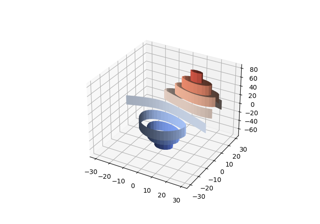# matplotlib.colors.LinearSegmentedColormap¶

class matplotlib.colors.LinearSegmentedColormap(name, segmentdata, N=256, gamma=1.0)[source]

Colormap objects based on lookup tables using linear segments.

The lookup table is generated using linear interpolation for each primary color, with the 0-1 domain divided into any number of segments.

Create colormap from linear mapping segments

segmentdata argument is a dictionary with a red, green and blue entries. Each entry should be a list of x, y0, y1 tuples, forming rows in a table. Entries for alpha are optional.

Example: suppose you want red to increase from 0 to 1 over the bottom half, green to do the same over the middle half, and blue over the top half. Then you would use:

cdict = {'red':   [(0.0,  0.0, 0.0),
(0.5,  1.0, 1.0),
(1.0,  1.0, 1.0)],

'green': [(0.0,  0.0, 0.0),
(0.25, 0.0, 0.0),
(0.75, 1.0, 1.0),
(1.0,  1.0, 1.0)],

'blue':  [(0.0,  0.0, 0.0),
(0.5,  0.0, 0.0),
(1.0,  1.0, 1.0)]}


Each row in the table for a given color is a sequence of x, y0, y1 tuples. In each sequence, x must increase monotonically from 0 to 1. For any input value z falling between x[i] and x[i+1], the output value of a given color will be linearly interpolated between y1[i] and y0[i+1]:

row i:   x  y0  y1
/
/
row i+1: x  y0  y1


Hence y0 in the first row and y1 in the last row are never used.

LinearSegmentedColormap.from_list

Static method; factory function for generating a smoothly-varying LinearSegmentedColormap.

static from_list(name, colors, N=256, gamma=1.0)[source]

Create a LinearSegmentedColormap from a list of colors.

Parameters
namestr

The name of the colormap.

colorsarray-like of colors or array-like of (value, color)

If only colors are given, they are equidistantly mapped from the range $$[0, 1]$$; i.e. 0 maps to colors and 1 maps to colors[-1]. If (value, color) pairs are given, the mapping is from value to color. This can be used to divide the range unevenly.

Nint

The number of rgb quantization levels.

gammafloat
reversed(name=None)[source]

Return a reversed instance of the Colormap.

Parameters
namestr, optional

The name for the reversed colormap. If it's None the name will be the name of the parent colormap + "_r".

Returns
LinearSegmentedColormap

The reversed colormap.

set_gamma(gamma)[source]

Set a new gamma value and regenerate colormap.

## Examples using matplotlib.colors.LinearSegmentedColormap¶Demonstrates plotting contour (level) curves in 3D using the extend3d option¶